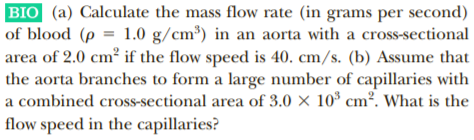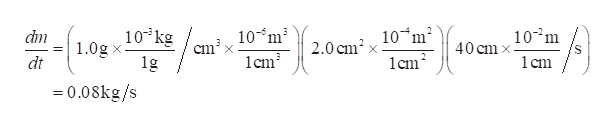# BIO (a) Calculate the mass flow rate (in grams per second)of blood (p = 1.0 g/cm³) in an aorta with a cross-sectionalarea of 2.0 cm² if the flow speed is 40. cm/s. (b) Assume thatthe aorta branches to form a large number of capillaries witha combined cross-sectional area of 3.0 × 10* cm². What is the|flow speed in the capillaries?

Question
7 viewshelp_outlineImage TranscriptioncloseBIO (a) Calculate the mass flow rate (in grams per second) of blood (p = 1.0 g/cm³) in an aorta with a cross-sectional area of 2.0 cm² if the flow speed is 40. cm/s. (b) Assume that the aorta branches to form a large number of capillaries with a combined cross-sectional area of 3.0 × 10* cm². What is the |flow speed in the capillaries? fullscreen
check_circle

Step 1

(a) The mass flow rate is

Step 2

Substitute the values in equation (1...help_outlineImage Transcriptionclose10 kg 1.0g x- 1g 10 m² 10-m 1cm? 10°m dm 2.0 cm? 40 cm x 1 cm 1cm? dt = 0.08kg/s fullscreen

### Want to see the full answer?

See Solution

#### Want to see this answer and more?

Solutions are written by subject experts who are available 24/7. Questions are typically answered within 1 hour.*

See Solution
*Response times may vary by subject and question.
Tagged in

### Physics